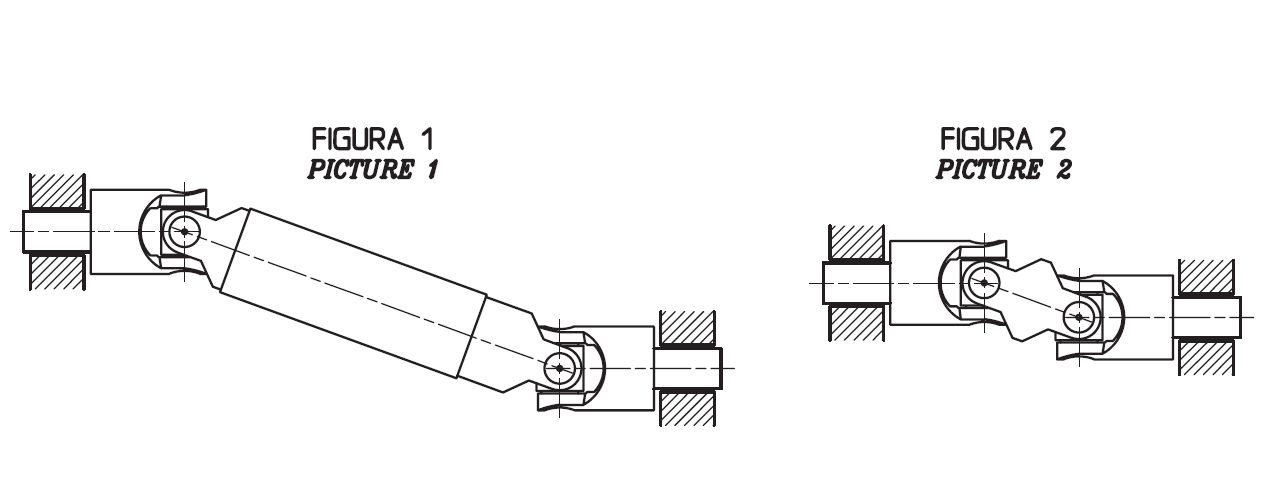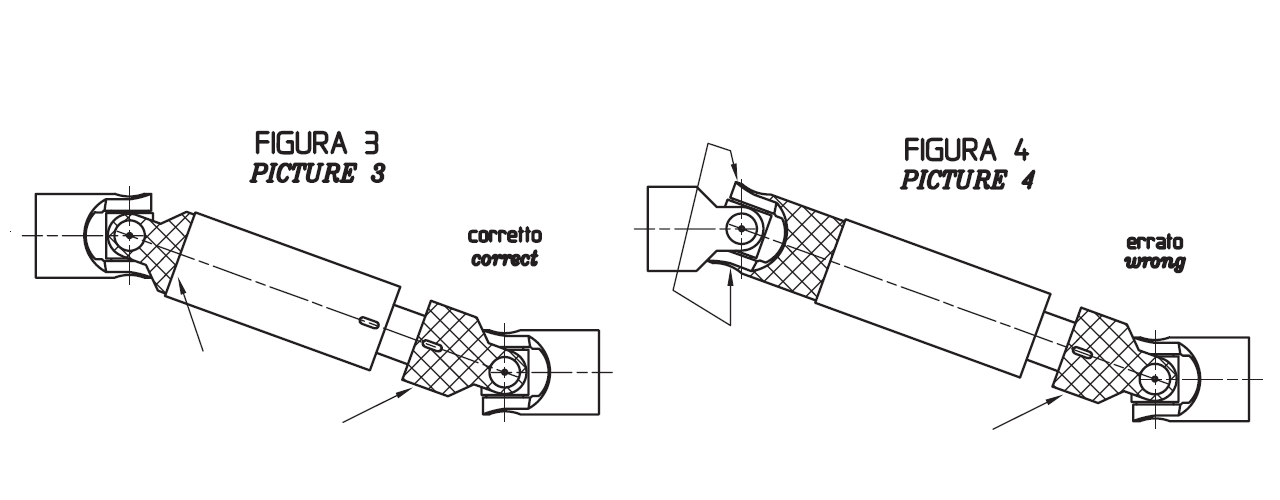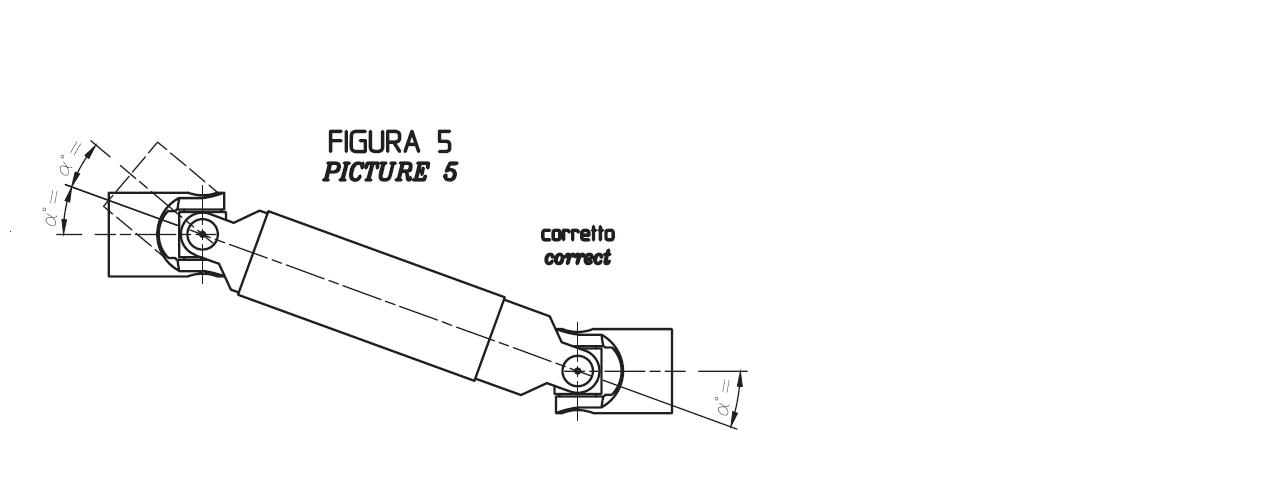# Technology and Support

## SELECTING CRITERIA

To have a homokinetic transmission, you have to fit either two apposite single joints (paying attention that the two central yokes lie on the same piane and the angles are equal) or a double joint.
The irregularity caused by the former articulation is cancelled by the latter. The overall length resulting from the coupling of the two single joints is even more reduced using a double joint. In other words, the double joint is to be considered as the shortest homokinetic transmission.

For low speed applications (max 1000rpm) joints with plain bearings (rubbing bearings) are suggested types S, G/GB.
They are able to support shock loads, irregular runnings and relatively high torques.
The working angles must be kept between 500 and 1000rpm.

For high rotation speeds, relatively low torques, motion reversals or wide angles, joints with needle roller bearings (type H) are to be preferred.
They can reach 4000rpm always relating to the angle α.

The joint capacity to transmit a regular torque at a constant load with no shocks, for a more or less long period, mainly depends on the number of revolutions per minute and the inclination angle α of the two axes.
The diagrams below have been conceived on the base of this criterium.
Each curve corresponds to the joint size (outside diameter «D») and represents the torque that the joint can transmit depending on speed and working angle α.
The diagrams can be directly read if angle (α) is 10°. For wider angles, torques are reduced, therefore these are to be corrected using the correction values (F) relating to the angle shown in the table.

IMPORTANT: Diagrams' values are merely indicative and are referred to the single joints only. When chosing a double joint, you have to consider that this one can transmit a torque about 10% lower than the same sized single joints. Each application has its own particular motion characteristics, such as: shock loads, motion reversals, connected masses, kind of starting, presence of elastic joints, stops and starts, etc, that have to be considered by the user when chosing the joint.

#### Diagram for JOINTS S-G

Example:

• Power: 0,65 KW
• R.P.M.: 230
• With working angle α 10° Value F=1 we get point P. Torque = 27 Nm corresponding to joint size <<D>> = 25/26 mm. = Types 04S, 04G, 1GB
• With working angle α 30° Value F=0,45 (Kw 0,65 : 0,45 = 1,44 Kw) we get point P1 Torque = 60 Nm corresonding to joint size <<D>> = 32 mm. = Mod. 1S, 1G, 3GB.

Consider that:         Torque in Nm = 9550   x         Power (KW)
R.P.M.

Torque in Nm = 7020   x         Power (HP)
R.P.M.

#### Diagram for JOINTS H

Example:

• Power: 5,5 KW
• R.P.M.: 2300
• With working angle α 10° Value F=1 we get point P Torque = 23 Nm corresponding to joint size <<D>> = 28/29 mm. = Types 05H
• With working angle α 25° Value F=0,70 (Kw 5,5 : 0,70 = 7,85 Kw) we get point P1 Torque = 33 Nm corresponding to joint size <<D>> = 32 mm. = Types 1H, 3HB.

Consider that:         Torque in Nm = 9550   x         Power (KW)
R.P.M.

Mt. in Nm = 7020   x         N (HP)
R.P.M.

## Instruction for a correct fitting upa. To obtain a uniform rotary motion always use either two opposite single joints or one double join. The pillow blocks are to be positioned as close as possible to the joints (see PICTURES 1 and 2).b. When using two opposite single joints respct the allignment of the inside yokes. In extensible transmitions also pay attention than the little lines stamped tally (see PICTURES 3 CORRECT, PICTURE 4 WRONG).c. The joints angles α must be equal (see PICTURE 5).